# Logic Design - Statetable Simplification and Implementation with one-hot encoding

Hey it's me again! Today we will talk about a Statetable Simplification Method and afterwards we will implement the Sequential Circuit of our Example in one-hot encoding using D Flip Flops. So, without further do let's get started!

# Introduction:

If you remember from this post, Statetables are used like Truth Tables in Sequential Circuits. Those Statetables contain all possible transitions from one State to another. We have Inputs that are only Inputs, but also Inputs that are the Feedback from Outputs! In those so called Sequential Circuits we have Memory Circuits like D Flip Flops that let us have the Circuit synchronized using a clock! That way our Input gets passed/the Output changes only when a specific clock event occurs (mostly positive edge triggered).

So, why do we need to simplify our Statetable? The answer is simple, sometimes some States can be grouped into one bigger group that represents the same State (that's exactly what we will do). Grouping States together we make our Circuit simpler, cause then it contains less States and Transitions! So, our Method today will find all the unique States grouping States that are actually the same into a new State. That way our Statetable will now contain new States, that represent the unique States from our previous Statetable!

Let's now get into the Simplification-Implementation process, applying it on an specific example!

# Statetable Simplification Method:

Our Example Statetable will be the following: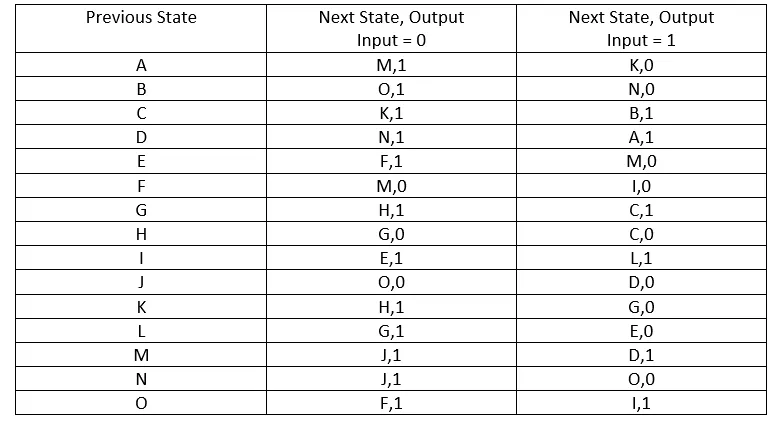Let's now simplify it step by step! The steps are the following:

1. Group States based on Output (1,0, 0,0, 1,1 etc.) inside of the Statetable
2. Create/Fill Staircase having each cell contain the State Groupings that must occur for it to be true. Remember States with different Output can't be Grouped, so we put X's
3. Start deleting the cells that have State Groupings inside that are deleted cells, until we can't delete anymore by putting X's inside
4. Create new States that represent the State Grouping and rewrite the Statetable

## Grouping of States based on Output:

We simply have to rewrite our Statetable spliting it up based on the Outputs. So, for example the first and second row have both 1 Output with X = 0 and 0 Output with X = 1 and so we put them continuously in our Statetable. When the Outputs change we will simply leave one row empty and continue filling.

So, our Statetable now looks like this: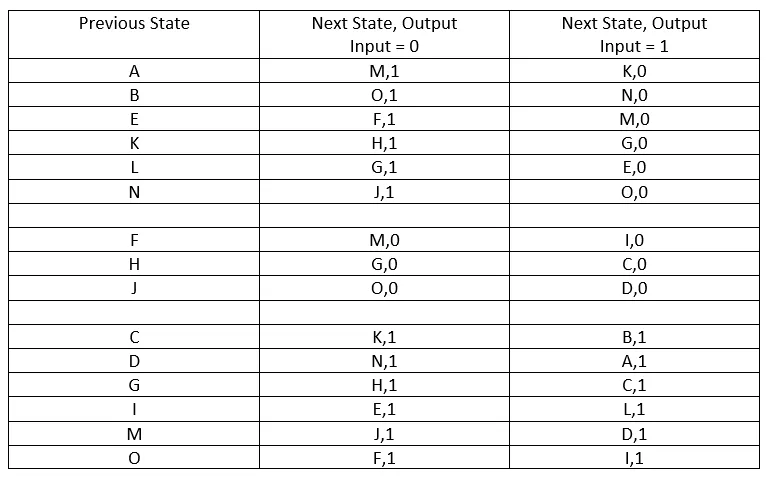## Create/Fill Staircase

The first "fill" is pretty simple. We have to put X's in all State Combinations that have different Outputs. We continue on checking the rows of the 2 States and writing down which States must be counterparts inorder to have the 2 first States be also counterparts or grouped.

So, our Staircase will look like this when starting out: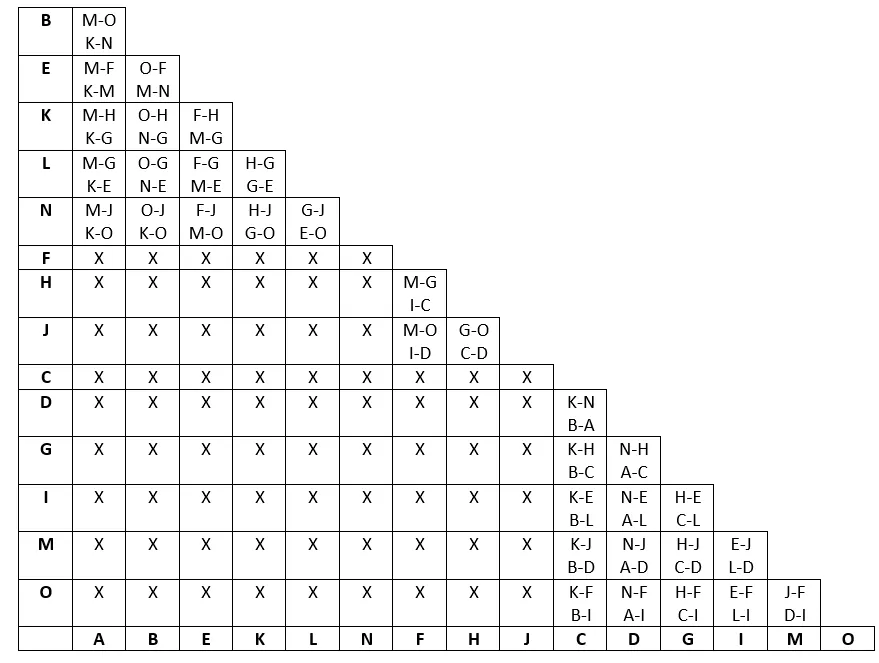You can see for example that A and F can't be grouped (X) cause they have different Outputs and so we have a X inside of this cell! A and B can be grouped, cause they have the same Output. But, for this to happen we will have to make M,O and K,N (that are the States for the specific Outputs 1 and 0 for the last to collumns) be counterparts. You can see that we wrote M-O and K-N inside of this A, B cell that means A and B are counterparts only when the other 2 conditions are met. The whole Staircase was filled using that logic.

## Deletion of False Cells:

But, not all of those Conditions inside are true! Groupings of different Outputs are declined directly and so we will delete any cell that contains even 1 counterpart-combination of States with different Outputs. We will also have to delete cells (make them false) when any of the 2 other State Combinations it contains are also false. A cell could contain counterpart-combinations of States with the same Outputs, but this Combinations can be an X inside of our Staircase (deleted previously, because of something being false) and so it again means false and we will have to delete this cell too. We will have to continue on this process until no more cells can be deleted!

Let's first delete the cells that contain combinations of States that have different Outputs.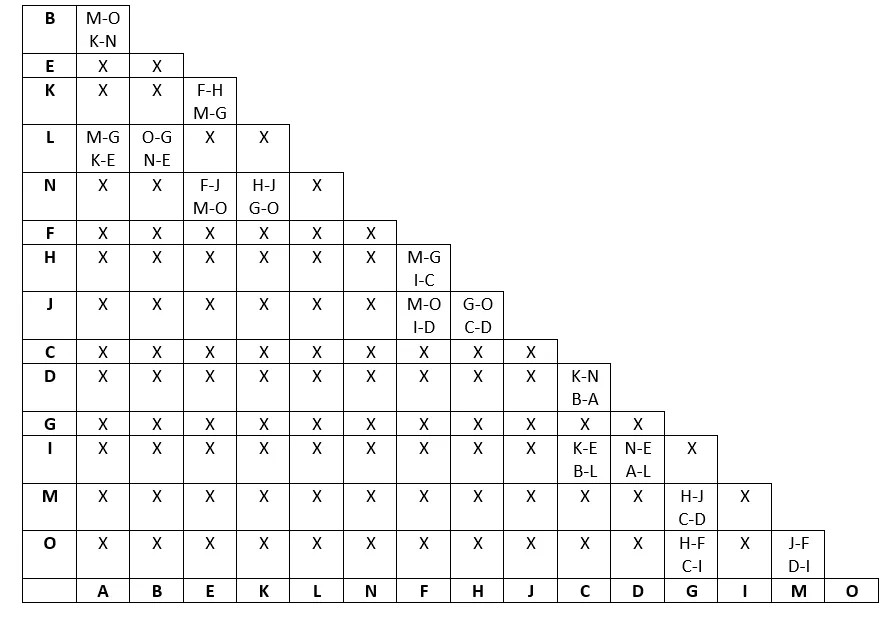Let's now delete the cells that contain cells that are possible combinations, but have an X inside of the Staircase and so become false as well, going into each of the cells one time in passes! But, by making one pass I saw that we already are finished! That could not happen in your case and you may have to check if any ot the combinations inside of a cell is false, until nothing more can be deleted!

So, the previous Staircase is also the last one!

## Create new States and rewrite the Statetable:

Checking the Staircase from the Final pass we can see that some counterpart-combinations remained. Those States will now be put inside of a new State.

So, for example A, B and L are all counterpart-combinations, cause our Staircase contains B-A, A-L and B-L and so can can make those be a new State called I for example that represents A, B and L.

Using this concept we end up with the following new States:

Ι: A-B-L, ΙΙ: K-N-E, ΙΙΙ: J-F-H, ΙV: C-D-I, V: G-M-O

Our new Statetable will now look like this: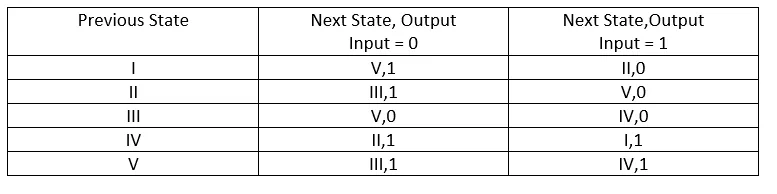We filled it pretty easily by going to the previous Statetable and checking any of the row's that contains a sub-State for each of the new States and replacing the "Output States" again with the new States!

# Implementation with one-hot encoding:

The implementation is pretty easy when we have this simplified version of our Statetable. One-hot encoding means that we will use as many binary digits/D Flip Flops as our States and have only one of the 1's be 1 for each State.

So, A -> 10000, B -> 01000, C -> 00100, D -> 00010, E -> 00001.

In our Example the Flip Flops A, B, C, D, E will correspond to the States I, II, III, IV and V of our Statetable!

We will fill a new Statetable that contains the Previous State, Input, Next State and Output representing the States with one-hot encoding and this Statetable looks like this:It's also pretty simple to get the functions out of there! We simply check only the rows with Output 1 and write the Minterm inside of the corresponding function DA, DB, DC, DD, DE for the D Flip Flop Input, only when the Next State corresponds to the specific D Flip Flop and having the previous State multiplied with X or X' depending on the Input!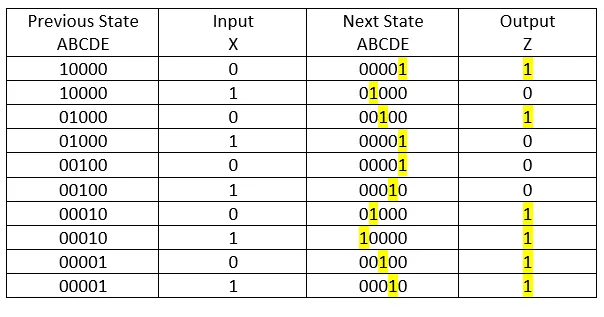So, in the first row for example the Previous State corresponds to A, the Next State to E and the Input X is 0 and so DE = AX' +... If we continue this process we will end up with the following Functions:

DA=DX, DB=AX+DX', DC=BX'+EX', DD=CX+EX, DE=AX'+BX+CX'

We also have to get the Output function in the same way and the Output Function Z looks like this:

Z=AX'+BX'+DX'+DX+EX'+EX = AX'+BX'+D+E

The Circuit implementation will be big, but it is also not quite difficult. We will have the Input X, 5 D Flip Flops with Probes and 1 Output Probe Z. The D Flip Flops need a Clock and we will have a Switch for the Input X and another Starting Switch to set the A as the first State. I will have the clock Frequency be low so that we have a big period and put a probe after the Clock to check the Clock's value. The rest of the Circuit will implementations of the Function we found out previously. Sometimes we prefer to put things like Multiplexers instead of those functions to make our Circuit even simpler, but I will implement those functions using Gates just to show you how complicated a Circuit can get!

So, our Circuit looks like this after pressing Start(S) and setting A to the first State:Let's now try to take Route I, II, III, V, IV, I using the needed X values. We will have to switch our Input everytime to the right value. To go from I to II we need X = 1. To go from II to III we need X = 0. To go from III to V we again need X = 0. To go from V to IV we need X = 1. Lastly, to return from IV to I we need X = 1 again.

We start off with State AWe put Input X = 1 and go to State B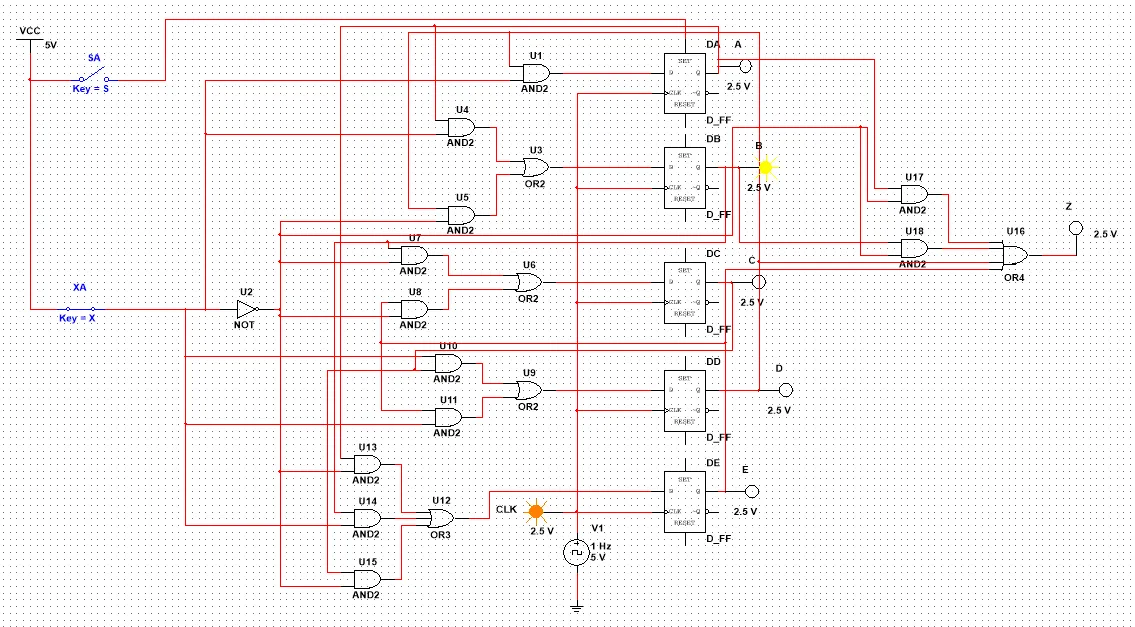We put Input X = 0 and get to State CWe keep X = 0 and get to State E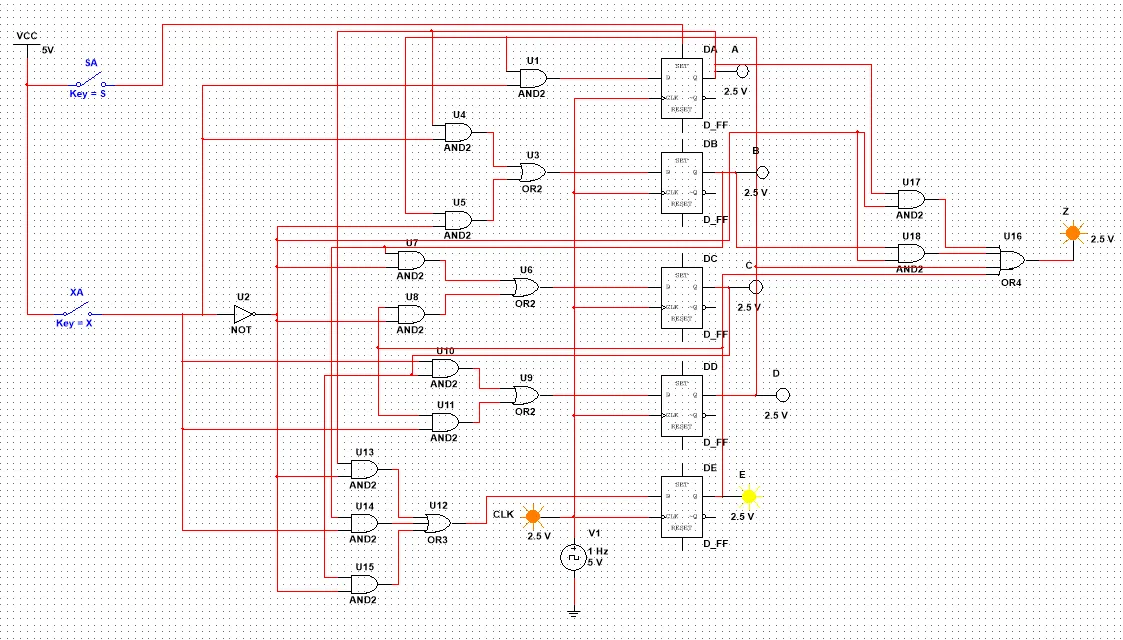We change the Input X and make it 1 and so transition to D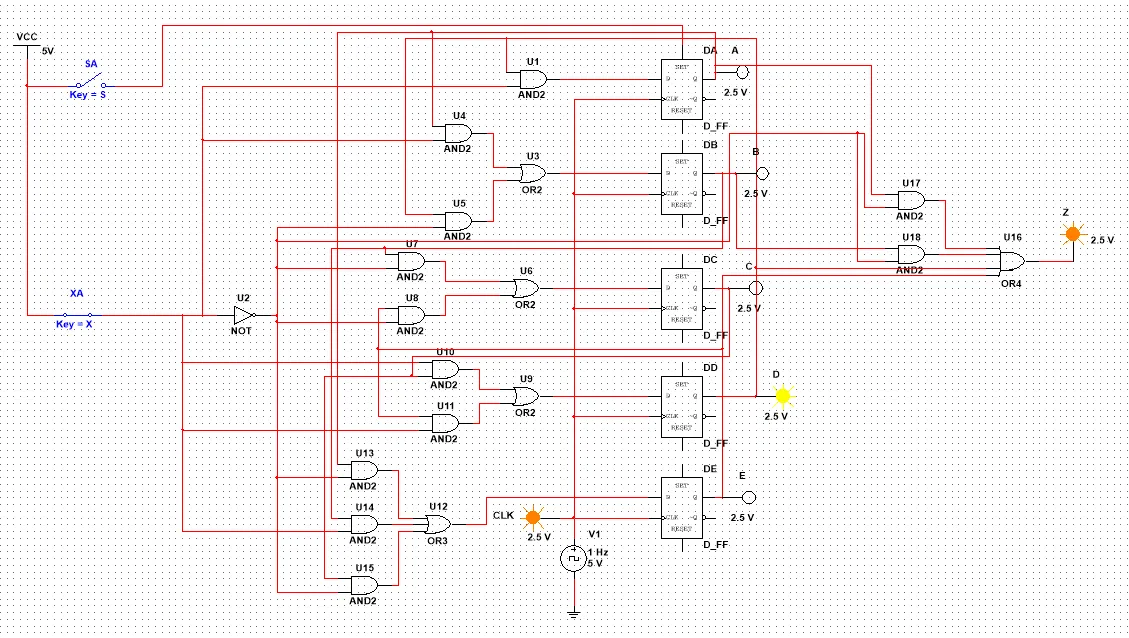We keep Input X as 1 and transition back to A!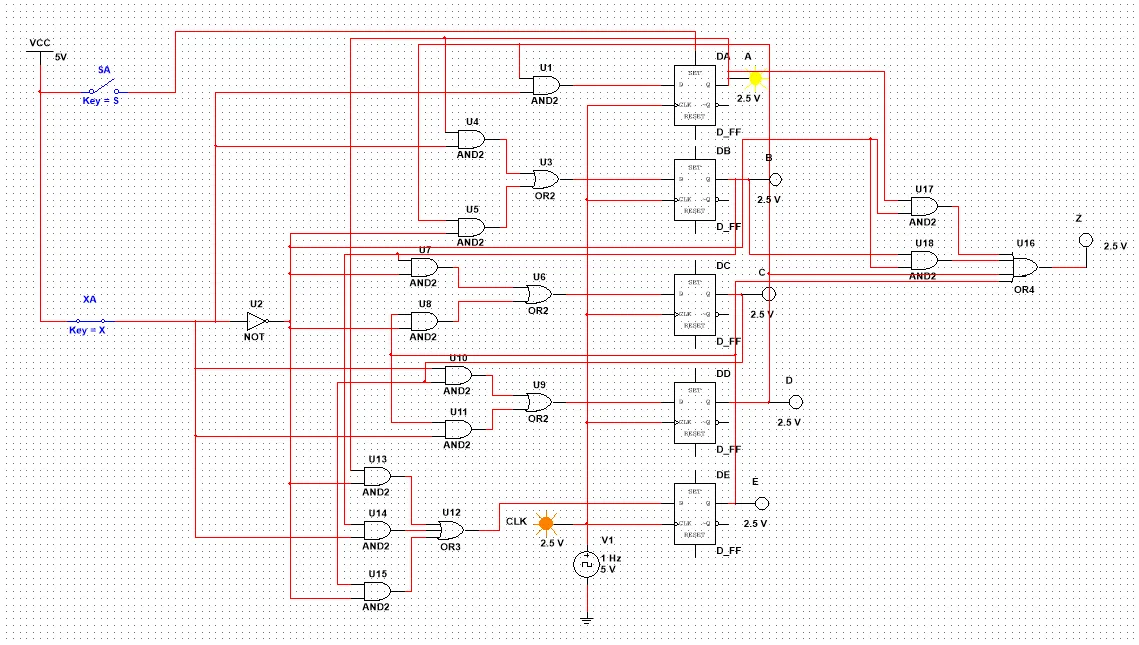We did it! It was not so difficult! And this is actually it!

Hope you enjoyed this post!

Next time in Logic Design we will maybe implement a Sequential Circuit using JK Flip Flops in Multisim (last Multisim post). I also have 1 more Logic Design Theory post for Binary Decision Diagrams and after that we can start getting into VHDL!

Bye!

H2
H3
H4
3 columns
2 columns
1 column
Join the conversation now